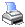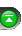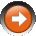﻿ GetBar()
 NinjaScript > Language Reference > Data > Bars > GetBar()Definition
Returns the bar index of the 1st bar from oldest to newest that matches the time stamp based on the DateTime parameter passed in. If the time stamp passed in is older than the 1st bar, a bar index of 0 is returned. If the time stamp is newer than the last bar, the last bar index is returned.

Method Return Value

An int value that represents the bar index value.

Syntax
Bars.GetBar(DateTime time)

Parameters

 time Time stamp to be converted to a bar index

Examples

 // Check that its past 9:45 AM if (ToTime(Time) >= ToTime(9, 45, 00)) {     // Calculate the bars ago value for the 9 AM bar for the current day     int barsAgo = CurrentBar - Bars.GetBar(new DateTime(2006, 12, 18, 9, 0, 0));       // Print out the 9 AM bar closing price     Print("The close price on the 9 AM bar was: " + Close[barsAgo].ToString()); }